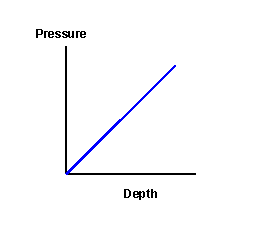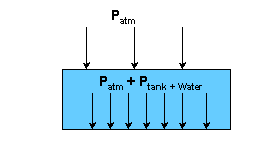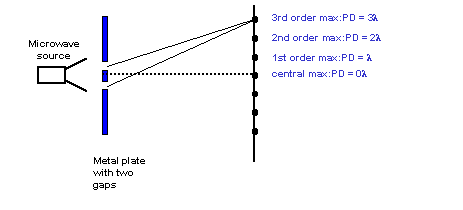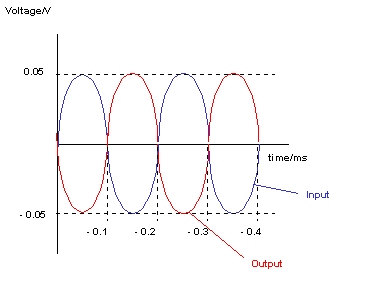# Solutions to SQA examination

## 2003

```Section A
1. D		11. D
2. E		12. E
3. A		13. C
4. D		14. B
5. A		15. B
6. C		16. B
7. E		17. D
8. C		18. A
9. B		19. E
10.E		20. A

Section B

21.a.i.  Vhorizontal=Vresultant x cosq
V=35xcos40o
Vhor = 26.81(m/s)

a.ii. Vvertical=Vresultant x sinq
Vver=35xsin40o
Vver = 22.5(m/s)

a.iii.Maximum height reached when the vertical component
of velocity (Vver) is 0m/s.
The vertical component of velocity calculated in part a.ii.
is the initial velocity (u) for the purpose of this calculation.

vver = 0(m/s)
uver = 22.5(m/s)
a = -9.8(m/s/s)
t = ?

v = u + at
t = (v-u)/a
t = (0-22.5)/-9.8
t = 2.29(s)

b. 	 Horizontal distance is calculated by multiplying the
total time of flight by the horizontal velocity, which
is constant throughout the flight.

Total time = [(2x2.29) + 0.48]s
ttotal = 5.06(s)
Vhor=26.81(m/s)

d = Vhor x ttotal
d = 26.81(m/s)x5.06(s)
d = 135.66(m)

22.a.  DPs = ms(vs-us)
DPs = 38(4.6-2.2)
DPs = 91.2(kgm/s)

b.	 Favgtc = DP
tc = DP/Favg
tc = 91.2/130
tc = 0.7(s)

c.  DPR = -DPS
DPR = -91.2(kgm/s)

DPR = mR(vR-uR)
-91.2 = 54(vR-2.2)
vR = 0.51(m/s)

d.   If kinetic energy is conserved the interaction is elastic.

Ekbefore = 1/2MRUR2 + 1/2MSUS2
Ekbefore = 0.5x54x2.22 + 0.5x38X2.22
Ekbefore = 222.64(J)

Ekafter = 1/2MRVR2 + 1/2MSVS2
Ekafter = 0.5x54x0.512 + 0.5x38x4.62
Ekafter = 409(J)

The gain in kinetic energy indicates that the collision is NOT ELASTIC.
The interaction is more like an explosion.

23.a.i.a.ii. Pliquid = rgh
Pliquid = 1000x9.8x0.25
Pliquid = 2450(Pa)

a.iii.As the depth increases the total pressure acting on the air inside
the tubing increases. Liquid enters the tube,compressing the air, until
the total pressure acting on the air inside the tube is equal to the
pressure of the air inside the tube.

b.	The pressure resulting from tank and water can
be calculated using:

P = F/A   where F is the weight of the tank and water.

F = wtotal = mtotalg
wtotal = (2.7x103+300)9.8
wtotal = 3000x9.8
wtotal = 29400(N)

A = 2.0x1.5 = 3.0m2

P = 29400/3
P = 9800(Pa)

Atmospheric pressure is pushing down on the tank. This means that
the total pressure acting on the surface that the tank is resting
on is the sum of the atmospheric pressure and the pressure resulting
from the weight of the tank.Ptotal = Ptank+water + Patmospheric
Ptotal = 9800 + 1.01x105
Ptotal = 1.108x105(Pa)

24.a.	P1 = 1.56x105Pa
T1 = (27+273)K = 300k

P2 = ?
T2 = (77+273)K = 350k

P1/T1 = P2/T2
P2 = P1T2/T1
P2 = 1.56x105x350/300
P2 = 1.82x105Pa

b.i.	P = I2Relement

I = V/Rtotal

I = 30/(0.5+1.5) = 30/2
I = 15A

P = 152x0.5
P = 112.5W

b.ii.	The output power of the element would be less.
This is because the current in the circuit would be
less because of the increase of the total series
resistance of the circuit.

Other explanations involving Vtpd are also valid.

25.a.i.	Y-gain = 5volts/div

a.ii.f = 1/T

T = 2.5ms = 2.5x10-3
f = 1/2.5x10-3
f = 400Hz

b.i.	VRMS = VPeak/SQRT2
VRMS = 12/1.414
VRMS = 8.49V

b.ii. E = 1/2(CVpk2)
E = 1/2(220x10-6x122)
E = 0.016J

b.iii.	The reading on the ammeter increases because the
current is directly proportional to the frequency.

b.iv.	The capacitor is constantly charging and discharging,
as the polarity of the supply voltage changes in this
ac circuit. The flow of charge during this process means
that the current in the circuit does not fall to zero.
In a dc circuit the capacitor charges and opposes the
flow of charge from the supply. When fully charged no
current flows in the circuit.

26.a.	 When the bridge is balanced:
R1/R2 = R3/R4

Where 	R1 = 5500W
R2 = RLDR
R3 = 330000W
R4 = 150000W

RLDR = (R1xR4)/R3
RLDR = (5500x150000)/330000)
RLDR = 2500W

b.i.	 MOSFET (n-channel)

b.ii.V1 = VX = 1.28V
V2 = VY = 1.50V

Vouput = (Rfeedback/R1)x(V2-V1)
Vouput = (22.5/1.5)x(1.5-1.28)
Vouput = 15x0.22
Vouput = 3.3V

b.iii.When water reaches the maximum level the beam of
light is not totally internally reflected. This means
that the light intensity incident on the LDR decreases,
increasing its resistance and increasing VX.
This decreases the output voltage from the differential
amplifier. When the output voltage falls below 2.0V
the MOSFET does not conduct and the current in the
solenoid falls to zero, closing the valve.

26.c.	The incident angle at the glass water boundary is less
than the critical angle so there is no total internal
reflection.

27.a.i.	The energy of the emitted photon is equal to the
energy difference between levels.The longest wavelength
radiation will have the lowest frequency and energy.

The smallest energy gap is beween E4 and E3

a.ii.	Ephoton = E3-E2
Ephoton = (-2.4x10-19)-(-5.6x10-19)
Ephoton = 3.2x10-19J

Ephoton = hf
f = Ephoton/h
f = 3.2x10-19/6.63x10-34
f = 4.83x1014Hz

b.i.	fair = fglass
fglass = 4.74x1014Hz

b.ii.	vair = fairlair
lair = vair/fair
lair = 3x108/4.74x1014
lair = 632.9nm

lglass = lair/n
lglass = 632.9/1.6
lglass = 395.6nm

28.a.i.	Maxima are produced when the path difference of the microwaves,
from each gap, to a point along AB is zero or a whole number of
wavelengths. This is because constructive interference occurs
at these points as the waves are in phase.

Minima are produced when the path difference of the microwaves,
from each gap, to a point along AB is l/2, 3l/2, 5l/2, 7l/2 etc.
This is because destructive interference occurs at these points
as the waves are P out of phase.

a.ii.PD = (766 - 682)mm
PD = 84mm

Third Max PD = 3l
3l = 84mm
l = 84/3
l = 28mm

b.i.	Gain = -Rfeedback/Rinput
Gain = -10kW/10kW
Gain = -1Voutput/Vinput = -Rfeedback/Rinput
Voutput = -Vinputx(Rfeedback/Rinput)
Voutput(peak) = -6x(3.0x103/2.0x103)
Voutput(peak) = -9V

b.ii.

29.a.i.	42He is is an alpha particle.

a.ii.	A = N/t

N = 7.2x105disintegrations
t = 2 minutes = 120s

A = 7.2x105/120
A = 6000Bq = 6kBq

b.i.	Half value thickness(t1/2)  =	thickness of barrier required to
reduce the count rate by half.

60counts/s -> 30counts/s requires 3cm
30counts/s -> 15counts/s requires 3cm

t1/2 = 3cm

b.ii.	The bar indicates the uncertainty in the average reading.
This results from the random nature of radioactive decay.

c.i.	Htotal = 6.4x10-5Sv

Alpha
Qa = 20
Ha = QaxDa
Ha = 20Da

Gamma
Qg = 1
Dg = 1.2x10-5Gy
Hg = QxDg
Hg = 1x1.2x10-5Sv
Hg = 1.2x10-5Sv

Htotal = Ha + Hg
6.4x10-5Sv = Ha + 1.2x10-5Sv
Ha = 6.4x10-5Sv - 1.2x10-5Sv
Ha = 5.2x10-5Sv

Ha = QDa
Ha = 20Da
20Da = 5.2x10-5Sv
Da = 5.2x10-5/20

Da = 2.6x10-6Gy = 2.6mGy

END OF QUESTION PAPER